# How to Calculate and Solve for Radial Flow Rate in Reservoir Fluid Flow | The Calculator Encyclopedia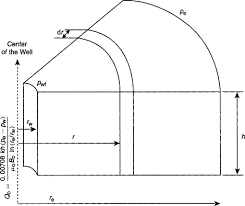The image above represents the radial flow rate.

To compute for the radial flow rate, eight essential parameters are needed and these parameters are External Pressure (Pe), Flowing Bottom-Hole Pressure (Pwf), Formation Thickness (h), Oil Viscosity (μo ), Permeability (k), Oil Formation Volume Factor (Bo), Drainage Radius (reand Well Bore Radius (rw ).

The formula for calculating the radial flow rate:

Qo = 0.00708kh[Pe – Pwf] / μo Bo In[re / rw]

Where;

Pe = External Pressure
Pwf = Flowing Bottom-Hole Pressure
h = Formation Thickness
μo = Oil Viscosity
k = Permeability
Bo = Oil Formation Volume Factor

Let’s solve an example;
Find the radial flow rate when the External Pressure is 14, Flowing Bottom-Hole Pressure is 21, Formation Thickness is 7, Oil Viscosity is 35, Permeability is 50, Oil Formation Volume Factor is 13, Drainage Radius is 26 and Well Bore Radius is 15.

This implies that;

Pe = External Pressure = 14
Pwf = Flowing Bottom-Hole Pressure = 21
h = Formation Thickness = 7
μo = Oil Viscosity = 35
k = Permeability = 50
Bo = Oil Formation Volume Factor = 13
re = Drainage Radius = 26
rw = Well Bore Radius = 15

Qo = 0.00708kh[Pe – Pwf] / μo Bo In[re / rw]
Qo = 0.00708 x 50 x 7 [14 – 21] / 35 x 13 In[26 / 15]
Qo = 0.00708 x 50 x 7 [-7] / 35 x 13 In[26 / 15]
Qo = 0.00708 x 50 x 7 [-7] / 35 x 13 In[1.73]
Qo = 0.00708 x 50 x 7 [-7] /35 x 13 x 0.55
Qo = 2.478 [-7] / 35 x 13 x 0.55
Qo = -17.346 / 250.27
Qo = -0.069

Therefore, the radial flow rate is -0.069 STB/day.

Nickzom Calculator – The Calculator Encyclopedia is capable of calculating the radial flow rate

To get the answer and workings of the radial flow rate using the Nickzom Calculator – The Calculator Encyclopedia. First, you need to obtain the app.

You can get this app via any of these means:

To get access to the professional version via web, you need to register and subscribe for NGN 1,500 per annum to have utter access to all functionalities.
You can also try the demo version via https://www.nickzom.org/calculator

Apple (Paid) – https://itunes.apple.com/us/app/nickzom-calculator/id1331162702?mt=8
Once, you have obtained the calculator encyclopedia app, proceed to the Calculator Map, then click on Petroleum under EngineeringNow, Click on Reservoir Fluid Flow under Petroleum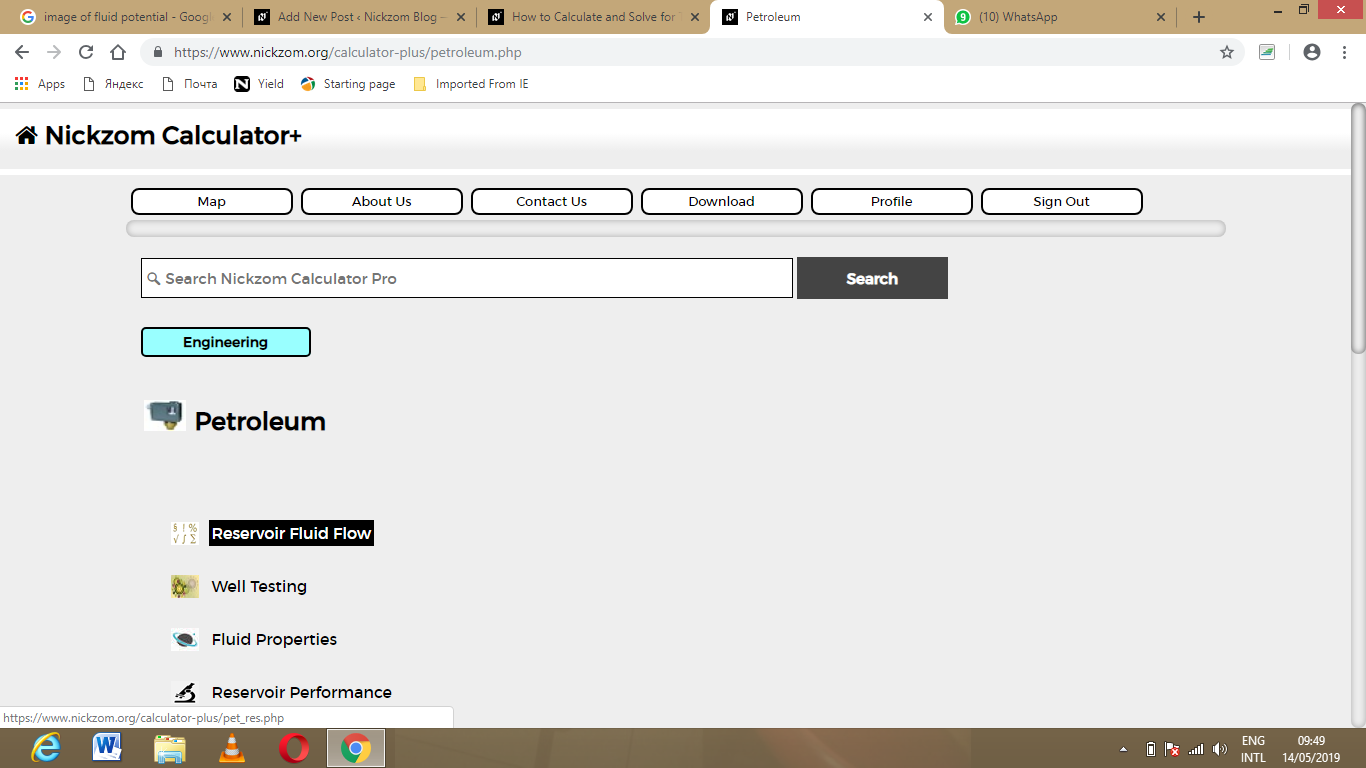Now, Click on Radial Flow Rate under Reservoir Fluid Flow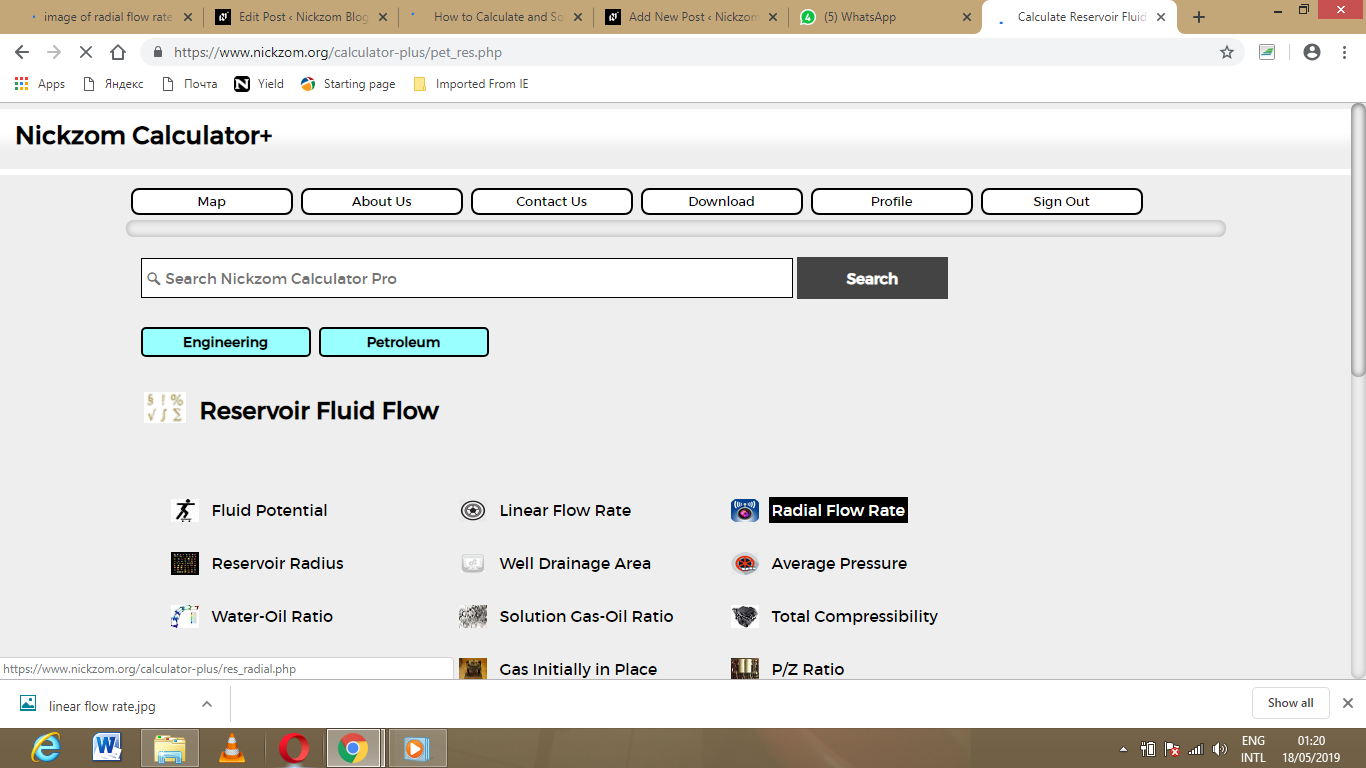The screenshot below displays the page or activity to enter your value, to get the answer for the radial flow rate according to the respective parameter which are the External Pressure (Pe), Flowing Bottom-Hole Pressure (Pwf), Formation Thickness (h), Oil Viscosity (μo ), Permeability (k), Oil Formation Volume Factor (Bo), Drainage Radius (reand Well Bore Radius (rw ).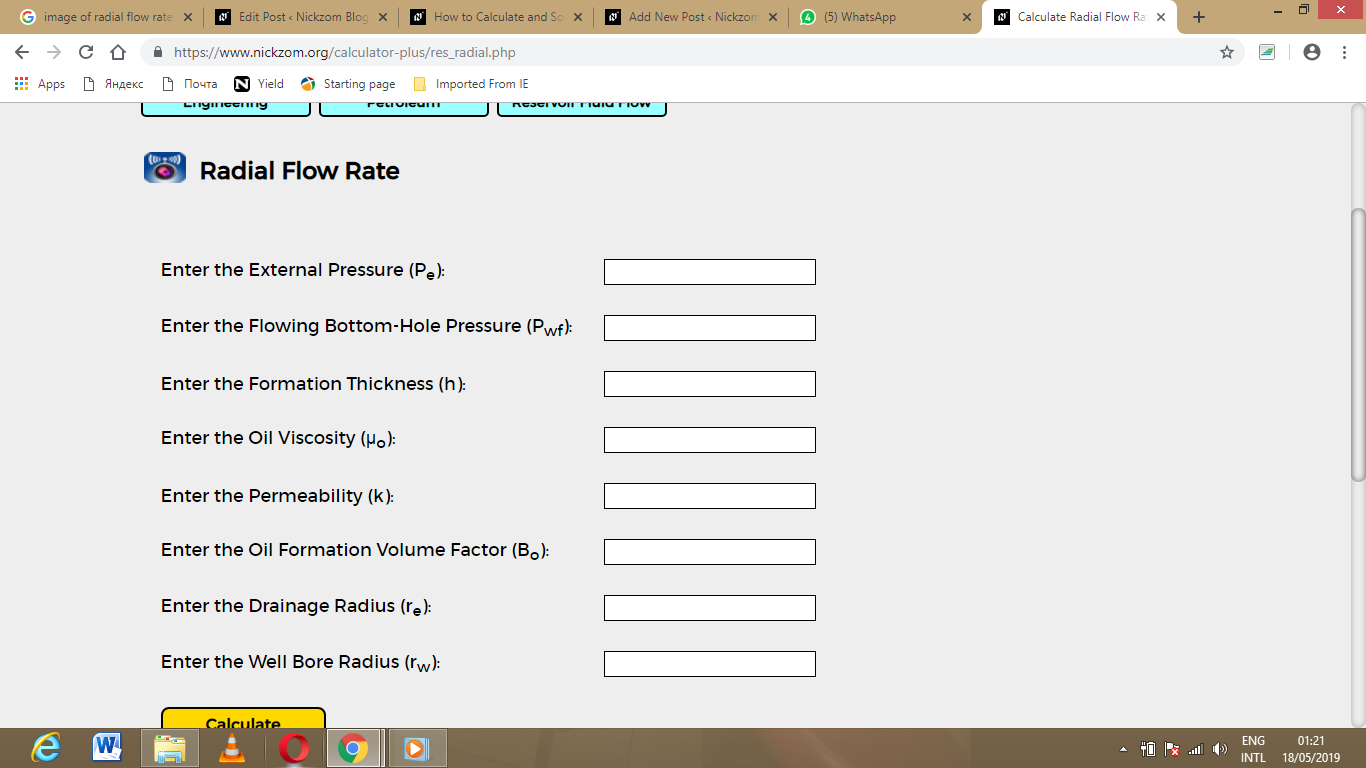Now, enter the value appropriately and accordingly for the parameter as required by the External Pressure (Pe) is 14, Flowing Bottom-Hole Pressure (Pwf) is 21, Formation Thickness (h) is 7, Oil Viscosity (μo ) is 35, Permeability (k) is 50, Oil Formation Volume Factor (Bo) is 13, Drainage Radius (re) is 26 and Well Bore Radius (rw ) is 15.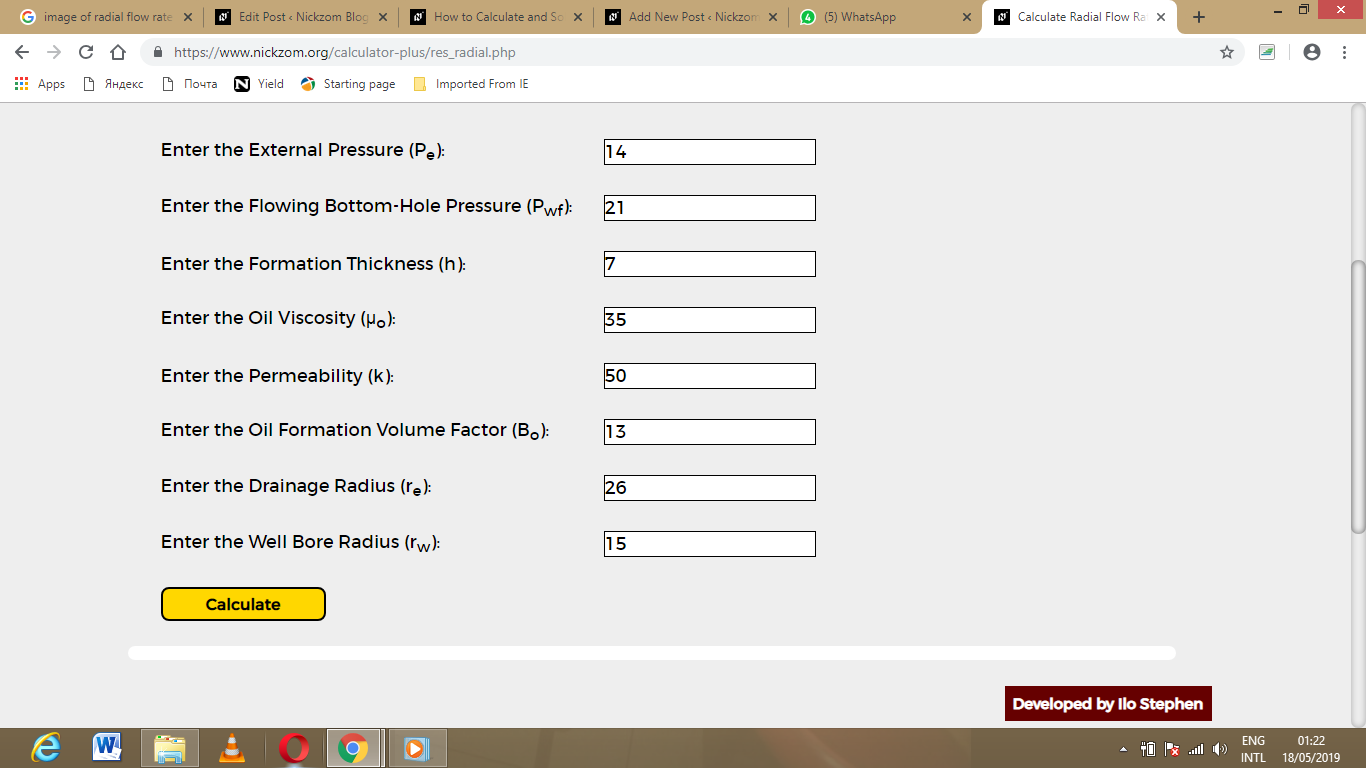Finally, Click on Calculate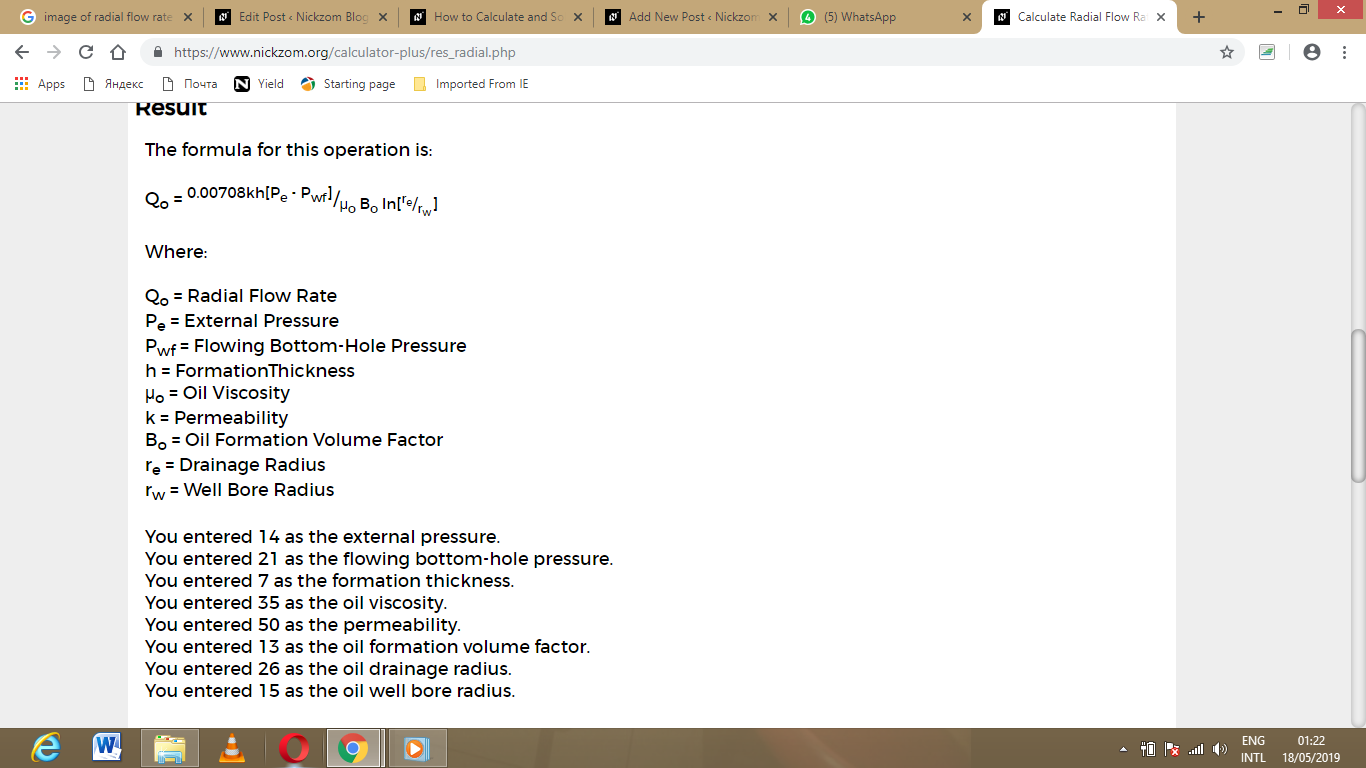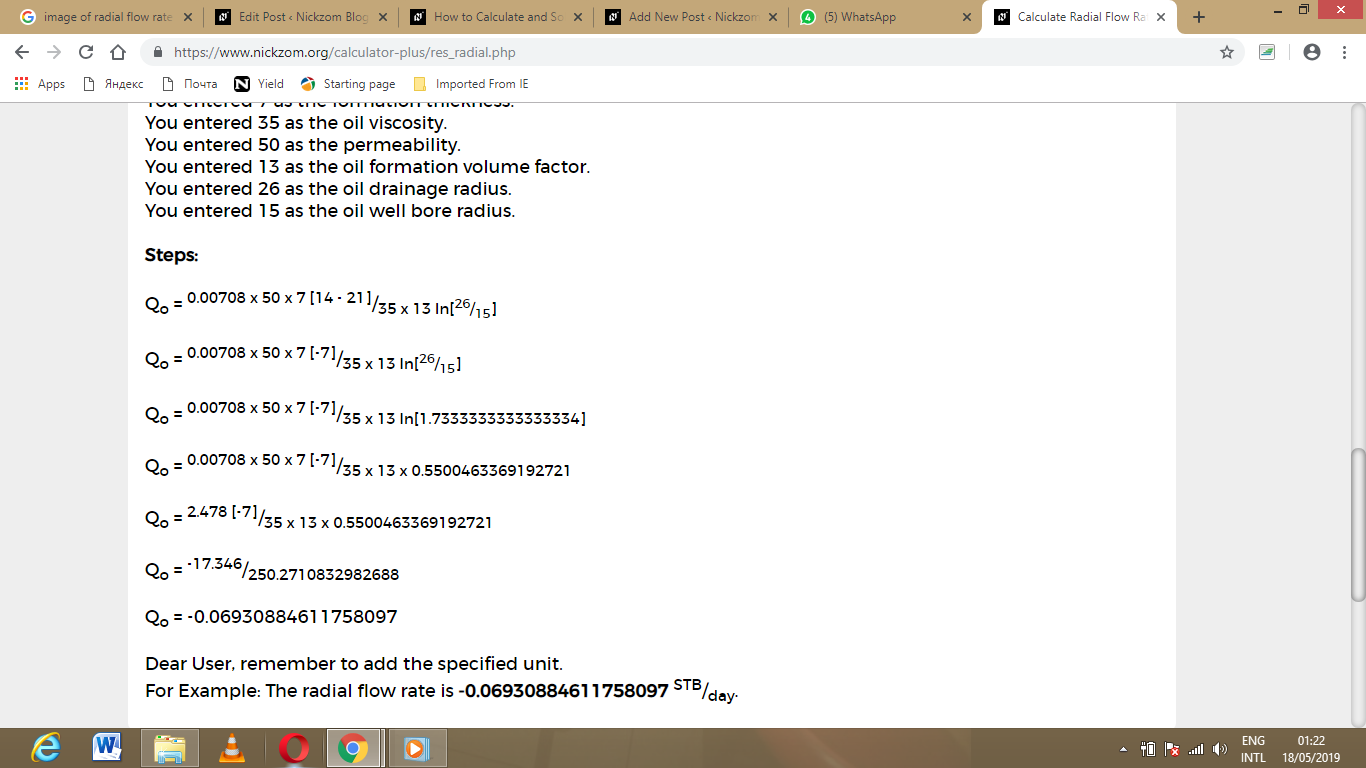As you can see from the screenshot above, Nickzom Calculator – The Calculator Encyclopedia solves for the radial flow rate and presents the formula, workings and steps too.